﻿ Simulation and Prediction of the Power Output and the Photocurrent for Photovoltaic SystemsPublications are Open
Access in this journal
Article Versions
Export Article
• Normal Style
• MLA Style
• APA Style
• Chicago Style
Research Article
Open Access Peer-reviewed

### Simulation and Prediction of the Power Output and the Photocurrent for Photovoltaic Systems

Kossi Kety, Apélété Komi Amou, Koffi Sagna , Yendoubé Lare, Kossi Napo
American Journal of Energy Research. 2017, 5(2), 41-50. DOI: 10.12691/ajer-5-2-3
Published online: August 24, 2017

### Abstract

We study in this paper, based on comparison already made in the literature concerning photovoltaic generator power models, the most optimal model applied to the operation of the photovoltaic generator of Sévagan (Togo). The comparison with the experimental data is carried out, which allowed us to verify the validity of the model. Finally, the influence of the characteristic parameters on the photovoltaic module ECO LINE LX-260P used to make the photovoltaic generator of the Sévagan dispensary (in Togo) is studied in order to predict the power production of the module according to the meteorological conditions(temperature-Irradiation). The comparison with the experimental data will be carried out in order to verify the validity of the model. To verify the validity of the model throughout the range of weather conditions, the process was done in two steps: on a sunny day and a cloudy day. A good agreement was observed with 95%, 97% and 99% correlation coefficients for cloudy, sunny days and the generator photocurrent simulation respectively. The results demonstrate an acceptable accuracy of the power model under different environmental conditions.

### 2. Materials and Methods

2.1. Materials and Experimental Method

The experimental equipment consists of an AHLBORN pyranometer type FL A613-GS, SN: 15111835/15, a thermometer serving as a temperature sensor, an ALMEMO datalogger and multimeters for measuring the current and voltage of ECO LINE LX-260P solar module.

Measurements were carried out at the Solar Energy Laboratory of the University of Lomé (LES-UL) on the ECO LINE LX-260P module as well as solar irradiation and ambient temperature. The same mesurements were all so done in Sévagan for the photovoltaic generator constitued by 11 modules ECO LINE LX-260P. All these measurements were made with an accuracy of 10-4. These data were automatically recorded using the ALMEMO control unit.

2.2. Power Models
2.2.1. Model 1: Benchmark Model 

This model was developed and validated experimentally by Lu Lin 3. It allows to determine the maximum power supplied by a photovoltaic module, for a sunshine G and a given module temperature with only four constant positive parameters, to be determined. The parameters a, b, c and d can be known experimentally, and a system of simple equations can be solved resulting in a sufficiently extended set of measurement points (Belhadj 8 and Jones 2).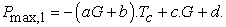(1)

With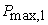the maximum power produced in Watt;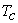: the temperature of the module that can be described by Equation 2. According to the experimental measurements carried out on a module (BP Solar 340), the constants a, b, c and d are 0.0002; 0.0004; 0.1007 and 0:1018 respectively. The NOCT of the module BP Solar 340 is 47∓2 °C; which allows us to find: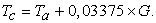(2)

So equation (1) becomes: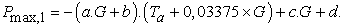(3)

2.2.2. Model 2: Input / Output Power Model

The power produced by a photovoltaic generator is estimated from the data of the global irradiation on an inclined plane, the ambient temperature and the data of the manufacturer for the photovoltaic module used. It is given by equation (4): (Fethi 4; R. Zeiba, 5)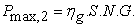(4)

With S: area of the module constituting the photovoltaic field,

N: Number of photovoltaic field modules, G: Irradiation on inclined plane,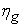: Instantaneous efficiency of the photovoltaic generator given by equation (5):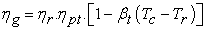(5): Reference efficiency of the modules under standard conditions,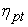: Efficiency of the tracking system of the maximum power point which is equal to 1 if a perfect system is used,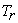: Reference temperature,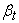: Experimentally determined temperature coefficient of yield.

It is defined as the variation of the efficiency of the module for a 1°C variation in the temperature of the cell. Typical values for this coefficient are between 0.004 and 0.006 (Fethi, 4; R. Zeiba 5).

2.2.3. Model 3: Simulation model of Borowy and Salameh

This model was developed by (Borowy and Salameh 1). The principle of the model is based on the equivalent circuit with one diode (Figure 1).

• Figure 1. Equivalent diagram of a solar cell: model with one diode

This model use the characteristics of the modules provided by the manufacturers. It therefore offers a very simple way of calculating the power produced by the PV modules. The formulas for calculating the maximum current intensity as well as the maximum voltage of the module under arbitrary conditions are given by the following relations (Lu Lin 7, Belhadj 8):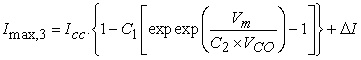(6)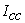: Short-circuit current of the module (in Ampere),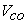: Open circuit voltage of the module (in Volt).

The parameters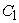and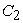are calculated by equations (7) and (8):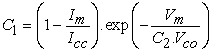(7)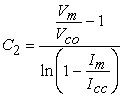(8)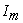: Maximum current of the module under standard conditions,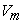: Maximum voltage under standard conditions

The maximum voltage under arbitrary conditions is (3):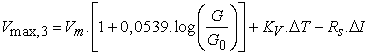(9)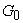: Solar irradiation under standard conditions (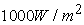),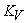: Temperature coefficient of open circuit voltage,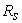: Series resistance of the module.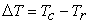(10)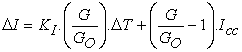(11)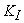is the temperature coefficient of the short-circuit current. The maximum power at the output of a module is determined by equation (12):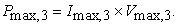(12)

For a number of modules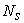in series and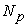in parallel, the maximum power produced is given by (equation 13):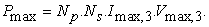(13)

2.2.4. Model 4: Jones and Underwood Simulation Model (Fill Factor Power Model)

The following model was developed by Jones and Underwood to calculate the maximum power delivered by a photovoltaic module.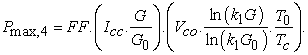(14)

The coefficient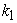is calculated by the following formula: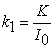(15)

Where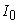is the inverse saturation current of the diode and K is a constant expressed in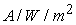defined by: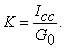(16)

FF is the fill factor defined by: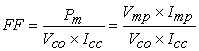(17)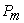is the maximum power of the module under standard test conditions.

2.2.5. Model of Prediction of the Power Produced by the Photovoltaic Generator of Sévagan

In the literature, several authors have carried out comparative studies between the different power models. The reference model is model 1 which was developed experimentally by Lu Lin 7. Lu Lin has checked and compared the model of Jones and Underwood and those of Borowy and Salameh 1. He found that the model of Borowy and Salameh gives a very good accuracy when solar irradiation and temperature are high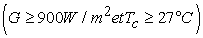. Belhadj Mohammed 8 then compared the three simplified power models to the reference model.

According to his results, the values delivered by the model input / output (Model 2) are in very good agreement with the reference model. In addition, the error is linear as a function of temperature and irradiation, this error does not exceed 0.5% in all cases (Kashif Ishaque 9). The input / output model was therefore used to estimate the power produced by the Sévagan photovoltaic generator.

2.3. Model to Describe the Behavior of Photovoltaic Cell

A photovoltaic cell is an optoelectronic component made of semiconductor materials doped differently: type P and type N. It transforms solar light into electricity by photovoltaic effect. The models used to describe the behavior of a real photovoltaic cell are the one-diode and two-diode models. The one-diode model is easy to implement but less accurate than the two diode model. The iterative method described in the work of Villalva (M. Villalva 10) is the best. However, its accuracy deteriorates for low irradiations, particularly in the vicinity of the open circuit voltage (Kashif Ishaque 11). The introduction of the second diode in the circuit increases the number of parameters to be determined: the inverse saturation current and the ideality factor of the second diode are added to the existing parameters. The determination of all parameters of the model constitutes the major problem. For the two-diode model, the Levenberg-Merquardt fitting technique is applied to construct the I-V curve, (Ja Gow 10). According to the work of (S. Chowdhury 11), an equivalent Thevenin circuit is used to estimate the model parameters, whereas according to Hovinen (Anssi Hovinen 12), the parameters are calculated as a function of the series resistance. However, in all these techniques, (Hovinen 12; J. Hyvarinen 13), several new additional coefficients are introduced into the equations. In addition, difficulties arise in determining the initial values of the parameters. Another approach to describe the two-diode model is to examine its physical characteristics such as electrons defect coefficient, lifetime of minority carriers, carrier intrinsic density, and other semiconductor parameters. The most important work in this field is the method of decomposing the irradiation of the solar cell (J. Hyvarinen 13) (the irradiance decay cell analysis method), the zone of predominance of the diffusion current (Ken-ichi Kurobe 14), the two-diode model modified (Kensuke Nishioka 15) and the modified three-diode model (Kensuke Nishioka 16). Although these models are useful for understanding the physical behavior of a solar cell, information on semiconductor parameters is not always available on the technical data sheets of commercially available photovoltaic cells. Moreover, in most of these works, the following values have been considered for ideality factors: n1 = 1 and n2 = 2. This assumption is widely used but is not always true. In view of these discussions, we can conclude that, although the two-diode model is preferable in terms of precision, its implementation requires more computation compared to the one-diode model, (Hovinen 12, J. Hyvarinen 13). Moreover, the modeling using the semiconductor approach as described in (Kensuke Nishioka 13) is not suitable because of the insufficient information on the technical data sheets. The method proposed by (Kashif Ishaque 9) has the advantage of simplifying the equation of current: only four parameters have been calculated. The equivalent electrical circuit of the model is shown in Figure 2.

• Figure 2. Equivalent electrical circuit of two diodes model

The output current is given by Equation (18):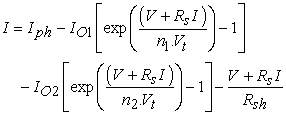(18)

Where I01 and I02 are the inverse saturation currents of diode D1 and diode D2 respectively. n1 and n2 represent the ideality factors of the diodes. The term containing I02 in equation 18 compensates for loss by recombination in the space charge zone as described in (Chih-Tang SAH 17). For the determination of the parameters, we rely on the model proposed by (Ishaque Kashif 9). The current is given by equation (22). A modification of equation 23 gives the inverse saturation currents I01 and I02 which are taken as equal.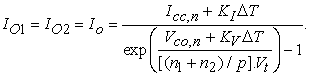(19)

Factors n1 and n2 represent the components of the diffusion and recombination current, respectively. In accordance with Shockley's theory of distribution, n1 must be equal to unity, (Chih-Tang SAH 17). The value of n2 can vary. A better compatibility is obtained between the proposed model and the experiment if n2 ≥ 1.2. Since (n1 + n2) / p = 1, it follows that p ≥ 2. This generalization eliminates the ambiguity in the choice of the values of n1 and n2. Equation (18) can be simplified in terms of p as: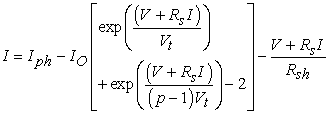(20)

Rs and Rsh are calculated simultaneously in a manner similar to the procedure proposed by (Ishaque Kashif 9). The maximum power calculated by the model I - V using equation (21), Pmax,m is equal to the maximum experimental power Pmax,e supplied by the manufacturer on the datasheet. Thus, Pmax,m = Pmax,e = Vm × Im gives us the equation 21 for the shunt resistance: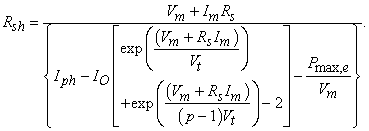(21)

The series resistor is initialized to zero and the value of the series resistance is set to zero. The shunt resistance initialy is given by equation (24). The iterative method of determining Rs and Rsh is represented by the flowchart of Figure 3; The Newton Raphson method will be applied to equation (20). The variable p can take any value greater than 2.2. Despite the high number of parameters, the model proposed by (Kashif Ishaque 11). only allows us to calculate four since I01 = I02 = I0; n1 = 1 and the value of p can be chosen arbitrarily, ie p ≥ 2.2.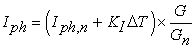(22)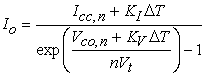(23)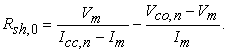(24)
• Figure 3. Flowchart of resolution of equation(20)

### 3. Results and Discussion

In this section, experimental data measured on site were compared to simulated power values. The parameters required for the calculation of the power are: the ambient temperature in the vicinity of the cells and the solar irradiation. These data are stored automatically by the ALMEMO control unit. For the experimental power values, voltmeter and an ampermeter were used to take the current and voltage measurements. The photo-voltage and photo-current values were taken every ten (10) minutes. The check was carried out for two typical days: a cloudy day (first day: Saturday 03 December 2016) and clear sky conditions with a few cloudy passages (day 2: Sunday 04 December 2016). In each case, the input / output model was compared to the experimental data. This allowed us to verify the validity of the model in the full range of weather conditions. At least 60 values for each of the clear sky and cloudy conditions were used to verify the simulation model. The comparison of the results is facilitated by the implementation of a linear correlation coefficient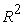given by equation (25) (Whei Zhou ):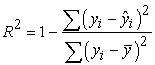(25)

With: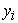represents the measured data of the current of the photovoltaic generator;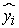is the current value predicted by the simulation model;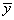is the arithmetic mean of the measured data.

3.1. Model Check: Case of Cloud Conditions

We present in Figure 4 the profiles of the measured and simulated values of the output power of the Sévagan photovoltaic generator in the case of a cloudy day.

• Figure 4. Comparison between the measured and simulated output power values for a cloudy day

We observe that the simulated power follows quite well the trend of the measured values. However, the observed deviations are due to a poor estimation of the temperature value of the cells by the model. The phenomenon of ambient air convection, thermal inertia and / or poor location of the thermal sensor may cause a delay between the measured ambient temperature used to predict the temperature of the cell and its actual temperature. We can emphasize that the values of the photocurrent and the voltage delivered by the photovoltaic generator are not measured simultaneously. Also, an increase in the error on the measured power, due to the indirect measurement by product I × V.

We also present on (Figure 5) the correlation between the simulated values and the measured values of the power.

• Figure 5. Correlation between measured and simulated power values (cloud conditions)

We see clearly that the calculated values vary linearly with the experimental data. Only slight differences were observed. The red line corresponds to the points where the calculated values coincide with the experimental values. The correlation coefficient thus calculated gives us a value of 0.95.

In the work of (Whei Zhou 18) correlation curves were plotted for four typical cloudy days of March, June, September and December. They obtained a linear correlation coefficientof 0.96.

3.2. Model Check: Clear Sky Day with Only a Few Cloudy

The data from our second measurement day in Sévagan were used to check the power model. We show in Figure 6 the profiles of the measured and simulated values of the output power. We find a good agreement of the simulation model with the experimental values. The deviations observed in this case are smaller compared to the cloudy day. Figure 7 shows the correlation between the measured values and the theoretical values. The correlation is linear with a correlation coefficient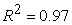. This correlation coefficient is higher than that obtained for the first cloudy day. This difference can be explained by a greater error on the estimated value of the temperature of the modules under cloudy conditions. Indeed, the rapid changes in the solar irradiation caused by the cloudy passages cause a sudden change in the ambient temperature. Given the thermal inertia of the thermometer used to measure the temperature, there may be discrepancies between the recorded value of the temperature and its instantaneous actual value.

This result is in agreement with that obtained in the literature. Our results are in agreement with those obtained by (Whei Zhou 18) in the case of clear sky days. He obtained a correlation coefficient of 0.98.

In both cases, cloudy or sunny weather, the high values of the correlation coefficient demonstrate a good prediction of the performance of the generator by the simulation model.

• Figure 6. Comparison between the measured and simulated power values (clear sky conditions)
• Figure 7. Correlation between measured and simulated power values (Clear sky conditions in Togo)
3.3. Results for Photocurrent Prediction

The Figure 8 show the I-V and P-V curves of the Ecoline LX -260P module which pass exactly through the three experimental points provided by the manufacturer in the technical figure. These curves show the nonlinear relationship between intensity and the voltage of the module on the one hand, and on the other hand between the power and the voltage of the module. They make it possible to understand the operation of a photovoltaic module subjected to a given illumination and a given operating temperature.

• Figure 8. Curves I - V and P - V adjusted to the three remarkable points: case of the model with two diodes

The following figures (Figure 9 and Figure 10) show the comparative curves of the simulated and experimental values for different ranges of solar irradiation. These comparisons have been made for the two diode models of the photovoltaic cell. Since solar irradiation and temperature at the module's neighbors are not constant, an average of these magnitudes over the duration of the experiment was considered in the model validation program.

• Figure 9. Theoretical and experimental I-V and P-V curves for G=310W/m²; T=33°C
• Figure 10. Theoretical and experimental I-V and P-V curves for G=850W/m²; T=35°C

In general, good agreement is observed between the experimental curves and the simulation results. However, more or less pronounced deviations are observed. These deviations can be explained by:

- Variation of the luminous flux received by the modulus during an experiment. The light flow received by the module greatly influences the photocurrent. This problem can be solved in the laboratory using either a model of characterization of the photovoltaic modules as described in the works of (Bertrand Gelis 19), or a solar simulator that fixes the illumination per unit area received by the photovoltaic module.

- Incorrect estimation of the operating temperature of the cells using the ambient temperature

- The phenomenon of air convection.

- The thermal inertia of the temperature sensor used may cause a delay between the ambient temperature used to predict the operating temperature of the cells and the actual temperature. Characterization models have a temperature probe to directly measure the temperature of the cells and thus avoid this problem.

- The performance of the module under real conditions: often the efficiency of the modules under actual conditions of use does not correspond to those provided by the manufacturer.

Presentation of the irradiation and temperature profiles of the Sévagan site and validation of the model

The final objective is to verify whether the model was able to predict the performance of the photovoltaic system. To validate the model, measurements were made over three days (from 03 to 05 December 2016). The total sunshine and the temperature in the vicinity of the modules recorded for these days are shown in Figure 11. The solar irradiation increases from the morning to reach a maximum at the solar noon before decreasing again until canceling out at nightfall. The first day of measurement was a very cloudy day with short periods of high solar irradiation. The solar irradiation at Sévagan can exceed; During the three days a maximum of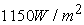was reached on the third day at 12h33min. The ambient temperature can drop to about 23.51°C at night, but during the day it depend on the level of sunshine and climatic conditions it can rise between 40 and 45° C.

• Figure 11. Variation of solar radiation and ambient temperature in the vicinity of the modules for three days at the Sévagan site

These types of temperature and solar irradiation profiles have been recorded by several authors, in particular (R. Merahi 20 and R. Shenni 21). The data were recorded at the Renewable Energy Research Center (CRAER) of the University of Nouakchott, in Mauritania over three days in August (R. Shenni 21). The temperature change over the three days showed a maximum temperature of 39 ° C on the third day. The maximum irradiation on this site during these three days did not exceed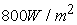. The simulation results of the photovoltaic generator were compared with the values measured in (Figure 12).

• Figure 12. Comparison of measured and simulated photocurrent values

We find a good agreement for the sunny day with only a few cloudy passages (second day). On the other hand, discrepancies are observed for the cloudy days. These deviations can also be explained by a poor estimation of the temperature value of the cells by the model. The phenomenon of ambient air convection, thermal inertia and / or poor location of the thermal sensor may cause a delay between the measured ambient temperature used to predict the temperature of the cell and its actual temperature.

Thus, the rapid changes in the solar irradiation caused by the cloudy passages cause a sudden change in the ambient temperature. Thus, there may be a phase shift between the stored ambient temperature value and its actual instantaneous value, and the values used in the model may not correspond to the actual values.

The correlation coefficients obtained for these three days are respectively: 0.9995; 0.9996 and 0.9963. We note that the sunniest day has the highest correlation coefficient. However, the high values of the correlation coefficient reflect the quality of the model proposed in this study to predict the performance of photovoltaic generators.

### 4. Conclusions

We present in this paper a list of the models of maximum power of a photovoltaic generator encountered in the literature. Simplified models of maximum power are very useful in practical applications as in the study of photovoltaic systems integrated in the building. On the basis of the work carried out by Lu Lin 7 and Belhadj Mohammed 8, the input / output model is used to predict the maximum output power of the Sevagan photovoltaic generator in Togo. To verify the validity of the model throughout the range of weather conditions, the verification was done in two steps: on a sunny day and a cloudy day. A good agreement was observed with 95%, 97% and 99% correlation coefficients for cloudy, sunny days respectively and the generator photocurrent simulation. The results demonstrate an acceptable accuracy of the power model under different environmental conditions.

### Acknowledgements

We present all our acknowledgments to the German Cooperation for the donation and installation of a photovoltaic generator from the ECO LINE LX-260P module at the Sévagan clinic and this, in association with the University of Lomé’s Solar Energy Laboratory.

### References

  Bogdan S Borowy, Ziyad M Salameh, 1996. Methodology for optimally sizing the combination of a battery bank and PV array in a wind/PV hybrid system. IEEE transactions on energy conversion, 11(2): 367-375. In article View Article  A.D. Jones et C.P. Underwood, 2002. A modelling method for building-integrated photovoltaic power supply. Building Services Engineering Research and Technology, 23(3): 167-177. In article View Article  Lin Lu et HX Yang, 2004. A study on simulations of the power output and practical models for building integrated photovoltaic systems. Journal of solar energy engineering, 126(3): 929-935. In article View Article  Fethi Benyarou, 2015. Conception assistée par ordinateur des systèmes photovoltaïques Modélisation, dimensionnement et simulation. Thèse de doctorat. In article View Article  R Zieba Falama, A Dadjé, N Djongyang et SY Doka, 2016. A new analytical modeling method for photovoltaic solar cells based on derivative power function. Journal of Fundamental and Applied Sciences, 8(2): 426-437. In article View Article  Ahmed Yahfdhou, Abdel Kader Mahmoud, Issakha Youm, 2016. Evaluation and determination of seven and five parameters of a photovoltaic generator by an iterative method. arXiv preprint arXiv:1601.03257. In article View Article  Lin Lu et HX Yang, 2010. Environmental payback time analysis of a roof-mounted building integrated photovoltaic (bipv) system in hong kong. Applied Energy, 87(12):3625-3631. In article View Article  Belhadj Mohammed, 2008. Modélisation d’un système de captage photovoltaïque autonome. Mémoire de Magistère, Université de Bechar. In article  Kashif Ishaque, Zainal Salam et Hamed Taheri, 2011. Simple, fast and accurate two-diode model for photovoltaic modules. Solar Energy Materials and Solar Cells, 95(2):586-594. In article View Article  JA Gow et CD Manning. 1996. Development of a model for photovoltaic arrays suitable for use in simulation studies of solar energy conversion systems. In Power Electronics and Variable Speed Drives, 1996. Sixth International Conference on (Conf. Publ. No. 429), 69-74. In article View Article  S Chowdhury, GA Taylor, SP Chowdhury, AK Saha etYH Song.. 2007. Modelling, simulation and performance analysis of a pv array in an embedded environment. In Universities Power Engineering Conference, UPEC 2007. 42nd International, 781-785. In article View Article  Anssi Hovinen. 1994. Fitting of the solar cell iv-curve to the two diode model. Physica Scripta, 1994 (T54):175. In article View Article  Jaakko Hyvarinen et Juha Karila. 2003. New analysis method for crystalline silicon cells. In Photovoltaic Energy Conversion, 2003. Proceedings of 3rd World Conference on, volume 2, 1521-1524. In article View Article  Ken-ichi Kurobe et Hiroyuki Matsunami. 2005. New two-diode model for detailed analysis of multicrystalline silicon solar cells. Japanese journal of applied physics, 44(12R):8314. In article View Article  Kensuke Nishioka, Nobuhiro Sakitani, Ken-ichi Kurobe, Yukie Yamamoto, Yasuaki Ishikawa, Yukiharu Uraoka et Takashi Fuyuki. 2003. Analysis of the temperature characteristics in polycrystalline Si solar cells using modiﬁed equivalent circuit model. Japanese journal of applied physics, 42(12R):7175. In article View Article  Kensuke Nishioka, Nobuhiro Sakitani, Yukiharu Uraoka et Takashi Fuyuki. 2007. Analysis of multicrystalline silicon solar cells by modiﬁed 3-diode equivalent circuit model taking leakage current through periphery into consideration. Solar Energy Materials and Solar Cells, 91(13): 1222-1227. In article View Article  Chih-Tang Sah, Robert N Noyce et William Shockley. 1957. Carrier generation and recombination in pn junctions and pn junction characteristics. Proceedings of the IRE, 45(9):1228-1243. In article View Article  Wei Zhou, Hongxing Yang, Zhaohong Fang, 2007. A novel model for photovoltaic array performance prediction. Applied energy, 84(12): 1187-1198. In article View Article  Bertrand Gélis, Vincent Creuze, Christian Glaize, Franck Lecat et Vincent Thomas. 2013. Travaux pratiques de caractérisation de panneaux photovoltaïques. In CETSIS: Colloque sur l’Enseignement des Technologies et des Sciences de l’Information et des Systèmes, numéro 10ème, 001-006. In article View Article  R Merahi, R Chenni et M Houbes, 2010. Modélisation et simulation d’un module pv par matlab. Journal of Scientific Research N° vol, 1. In article View Article  R Chenni, M Makhlouf, T Kerbache A Bouzid, 2007. A detailed modeling method for photovoltaic cells. Energy, 32(9): 1724-1730. In article View ArticleThis work is licensed under a Creative Commons Attribution 4.0 International License. To view a copy of this license, visit http://creativecommons.org/licenses/by/4.0/